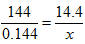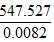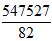Courses

# Decimal MCQ

## 20 Questions MCQ Test UPSC Prelims Paper 2 CSAT - Quant, Verbal & Decision Making | Decimal MCQ

Description
This mock test of Decimal MCQ for Quant helps you for every Quant entrance exam. This contains 20 Multiple Choice Questions for Quant Decimal MCQ (mcq) to study with solutions a complete question bank. The solved questions answers in this Decimal MCQ quiz give you a good mix of easy questions and tough questions. Quant students definitely take this Decimal MCQ exercise for a better result in the exam. You can find other Decimal MCQ extra questions, long questions & short questions for Quant on EduRev as well by searching above.
QUESTION: 1

Solution:
QUESTION: 2

Solution:
QUESTION: 3

### 0.005 ÷ 0.25 is equal to

Solution:
QUESTION: 4

36.45 ÷ 0.2 is equal to

Solution:
QUESTION: 5

2 ÷  5 is equal to

Solution:
QUESTION: 6

On simplifying 1.07 + 2.7 X 1.02 ÷ 1.6 we get

Solution:
QUESTION: 7

1 km is equal to

Solution:
QUESTION: 8

1 hg is equal to

Solution:
QUESTION: 9

A printer takes 0.012 seconds to print one alphabet. How long will he take to print the word ‘mathematics’?

Solution: It is just simple multiplication nothing else.
The word mathematics contains 11 words
according to ques each word will take exactly 0.012 second
So to print mathematics it will take 0.012×11 seconds that will be equal to 0.132 seconds. Therefore option C is correct. :-)
QUESTION: 10

700 m expressed in km is

Solution:
QUESTION: 11

Value of 1.1 x 0.1 is

Solution:
QUESTION: 12

Rs. 0.08 equals

Solution:
QUESTION: 13

3 kg 50 gm expressed as kg is

Solution:
QUESTION: 14

6 ÷  0.3 equals

Solution:
QUESTION: 15

0.213 ÷ 0.00213 = ?

Solution:
QUESTION: 16

1/0.04 is equal to

Solution:
QUESTION: 17

.009/? = .01

Solution:
QUESTION: 18

.04 x ? = .000016

Solution:
QUESTION: 19then value of x is

Solution:
QUESTION: 20

Ifthen value ofis

Solution: